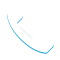### Introduction to Machine Learning Models Using IBM SPSS Modeler (V18.2) – 0A079G CR

Details

Course Code: 0A079G

Brand: DS&BA – SPSS

Category: Analytics

Skill Level: Basic

Duration: 16.00H

Modality: CR

Audience

• Data scientists
• Business analysts
• Clients who want to learn about machine learning models

Prerequisites

• Knowledge of your business requirements

Short Summary

This course teaches how to use machine learning models to predict categorical and continuous targets, to create natural groupings, and to find associations.

Overview

This course provides an introduction to supervised models, unsupervised models, and association models. This is an application-oriented course and examples include predicting whether customers cancel their subscription, predicting property values, segment customers based on usage, and market basket analysis.

Topic

Introduction to machine learning models
• Taxonomy of machine learning models
• Identify measurement levels
• Taxonomy of supervised models
• Build and apply models in IBM SPSS Modeler

Supervised models: Decision trees – CHAID
• CHAID basics for categorical targets
• Include categorical and continuous predictors
• CHAID basics for continuous targets
• Treatment of missing values

Supervised models: Decision trees – C&R Tree
• C&R Tree basics for categorical targets
• Include categorical and continuous predictors
• C&R Tree basics for continuous targets
• Treatment of missing values

Evaluation measures for supervised models
• Evaluation measures for categorical targets
• Evaluation measures for continuous targets

Supervised models: Statistical models for continuous targets – Linear regression
• Linear regression basics
• Include categorical predictors
• Treatment of missing values

Supervised models: Statistical models for categorical targets – Logistic regression
• Logistic regression basics
• Include categorical predictors
• Treatment of missing values

Supervised models: Black box models – Neural networks
• Neural network basics
• Include categorical and continuous predictors
• Treatment of missing values

Supervised models: Black box models – Ensemble models
• Ensemble models basics
• Improve accuracy and generalizability by boosting and bagging
• Ensemble the best models

Unsupervised models: K-Means and Kohonen
• K-Means basics
• Include categorical inputs in K-Means
• Treatment of missing values in K-Means
• Kohonen networks basics
• Treatment of missing values in Kohonen

Unsupervised models: TwoStep and Anomaly detection
• TwoStep basics
• TwoStep assumptions
• Find the best segmentation model automatically
• Anomaly detection basics
• Treatment of missing values

Association models: Apriori
• Apriori basics
• Evaluation measures
• Treatment of missing values

Association models: Sequence detection
• Sequence detection basics
• Treatment of missing values

Preparing data for modeling
• Examine the quality of the data
• Select important predictors
• Balance the data

Objectives

Introduction to machine learning models
• Taxonomy of machine learning models
• Identify measurement levels
• Taxonomy of supervised models
• Build and apply models in IBM SPSS Modeler

Supervised models: Decision trees – CHAID
• CHAID basics for categorical targets
• Include categorical and continuous predictors
• CHAID basics for continuous targets
• Treatment of missing values

Supervised models: Decision trees – C&R Tree

• C&R Tree basics for categorical targets
• Include categorical and continuous predictors
• C&R Tree basics for continuous targets
• Treatment of missing values

Evaluation measures for supervised models
• Evaluation measures for categorical targets
• Evaluation measures for continuous targets

Supervised models: Statistical models for continuous targets – Linear regression
• Linear regression basics
• Include categorical predictors
• Treatment of missing values

Supervised models: Statistical models for categorical targets – Logistic regression
• Logistic regression basics
• Include categorical predictors
• Treatment of missing values

Association models: Sequence detection
• Sequence detection basics
• Treatment of missing values

Supervised models: Black box models – Neural networks
• Neural network basics
• Include categorical and continuous predictors
• Treatment of missing values

Supervised models: Black box models – Ensemble models
• Ensemble models basics
• Improve accuracy and generalizability by boosting and bagging
• Ensemble the best models

Unsupervised models: K-Means and Kohonen
• K-Means basics
• Include categorical inputs in K-Means
• Treatment of missing values in K-Means
• Kohonen networks basics
• Treatment of missing values in Kohonen

Unsupervised models: TwoStep and Anomaly detection
• TwoStep basics
• TwoStep assumptions
• Find the best segmentation model automatically
• Anomaly detection basics
• Treatment of missing values

Association models: Apriori
• Apriori basics
• Evaluation measures
• Treatment of missing values

Preparing data for modeling
• Examine the quality of the data
• Select important predictors
• Balance the data

Tags :Call Now +27 72 266 2599